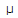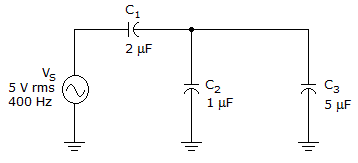# Electronics - Capacitors

### Exercise :: Capacitors - Filling the Blanks

1.

If a 5 V, 60 Hz voltage is applied to a 4.7F capacitor, the circuit current will be ______ .

 A. 0.886 mA B. 8.86 mA C. 8.86A D. 88.6A

Explanation:

No answer description available for this question. Let us discuss.

2.

___ capacitors have the highest values of capacitance.

 A. Electrolytic B. Ceramic C. Plastic-film D. Mica

Explanation:

No answer description available for this question. Let us discuss.

3.

The basic capacitor is made up of ___ that separates two conductive plates.

 A. a conductor B. a spacer C. a dielectric D. an electrode

Explanation:

No answer description available for this question. Let us discuss.

4.The total circuit reactance is ______ in the given circuit.

 A. 50B. 140C. 265D. 676Explanation:

No answer description available for this question. Let us discuss.

5.

A circuit whose output voltage is proportional to the rate of change of the input voltage is called a(n) ______ .

 A. differentiator B. integrator C. curve averager D. mixer